Want to share your content on R-bloggers? click here if you have a blog, or here if you don't.# Introduction

R is known to be a really powerful programming language when it comes to graphics and visualizations (in addition to statistics and data science of course!).

To keep it short, graphics in R can be done in two ways, via the:

1. `{graphics}` package (the base graphics in R, loaded by default)
2. `{ggplot2}` package (which needs to be installed and loaded beforehand)

The `{graphics}` package comes with a large choice of plots (such as `plot`, `hist`, `barplot`, `boxplot`, `pie`, `mosaicplot`, etc.) and additional related features (e.g., `abline`, `lines`, `legend`, `mtext`, `rect`, etc.). It is often the preferred way to draw plots for most R users, and in particular for beginners to intermediate users.

Since its creation in 2005 by Hadley Wickham, `{ggplot2}` has grown in use to become one of the most popular R packages and the most popular package for graphics and data visualizations. The `{ggplot2}` package is a much more modern approach to creating professional-quality graphics. More information about the package can be found at ggplot2.tidyverse.org.

In this article, we will see how to create common plots such as scatter plots, line plots, histograms, boxplots, barplots, density plots in R with this package. If you are unfamiliar with any of these types of graph, you will find more information about each one (when to use it, its purpose, what does it show, etc.) in my article about descriptive statistics in R.

# Data

To illustrate plots with the `{ggplot2}` package we will use the `mpg` dataset available in the package. The dataset contains observations collected by the US Environmental Protection Agency on fuel economy from 1999 to 2008 for 38 popular models of cars (run `?mpg` for more information about the data):

```library(ggplot2)
dat <- ggplot2::mpg```

Before going further, let’s transform the `cyl`, `drv`, `fl`, `year` and `class` variables in factor with the `transform()` function:

```dat <- transform(dat,
cyl = factor(cyl),
drv = factor(drv),
fl = factor(fl),
year = factor(year),
class = factor(class)
)```

# Basic principles of `{ggplot2}`

The `{ggplot2}` package is based on the principles of “The Grammar of Graphics” (hence “gg” in the name of `{ggplot2}`), that is, a coherent system for describing and building graphs. The main idea is to design a graphic as a succession of layers.

The main layers are:

1. The dataset that contains the variables that we want to represent. This is done with the `ggplot()` function and comes first.
2. The variable(s) to represent on the x and/or y-axis, and the aesthetic elements (such as color, size, fill, shape and transparency) of the objects to be represented. This is done with the `aes()` function (abbreviation of aesthetic).
3. The type of graphical representation (scatter plot, line plot, barplot, histogram, boxplot, etc.). This is done with the functions `geom_point()`, `geom_line()`, `geom_bar()`, `geom_histogram()`, `geom_boxplot()`, etc.
4. If needed, additional layers (such as labels, annotations, scales, axis ticks, legends, themes, facets, etc.) can be added to personalize the plot.

To create a plot, we thus first need to specify the data in the `ggplot()` function and then add the required layers such as the variables, the aesthetic elements and the type of plot:

```ggplot(data) +
aes(x = var_x, y = var_y) +
geom_x()```
• `data` in `ggplot()` is the name of the data frame which contains the variables `var_x` and `var_y`.
• The `+` symbol is used to indicate the different layers that will be added to the plot. Make sure to write the `+` symbol at the end of the line of code and not at the beginning of the line, otherwise R throws an error.
• The layer `aes()` indicates what variables will be used in the plot and more generally, the aesthetic elements of the plot.
• Finally, `x` in `geom_x()` represents the type of plot.
• Other layers are usually not required unless we want to personalize the plot further.

Note that it is a good practice to write one line of code per layer to improve code readability.

# Create plots with `{ggplot2}`

In the following sections we will show how to draw the following plots:

• scatter plot
• line plot
• histogram
• density plot
• boxplot
• barplot

In order to focus on the construction of the different plots and the use of `{ggplot2}`, we will restrict ourselves to drawing basic (yet beautiful) plots without unnecessary layers. For the sake of completeness, we will briefly discuss and illustrate different layers to further personalize a plot at the end of the article (see this section).

Note that if you still struggle to create plots with `{ggplot2}` after reading this tutorial, you may find the {esquisse} addin useful. This addin allows you to interactively (that is, by dragging and dropping variables) create plots with the `{ggplot2}` package. Give it a try!

## Scatter plot

We start by creating a scatter plot using `geom_point`. Remember that a scatter plot is used to visualize the relation between two quantitative variables.

1. We start by specifying the data:
`ggplot(dat) # data`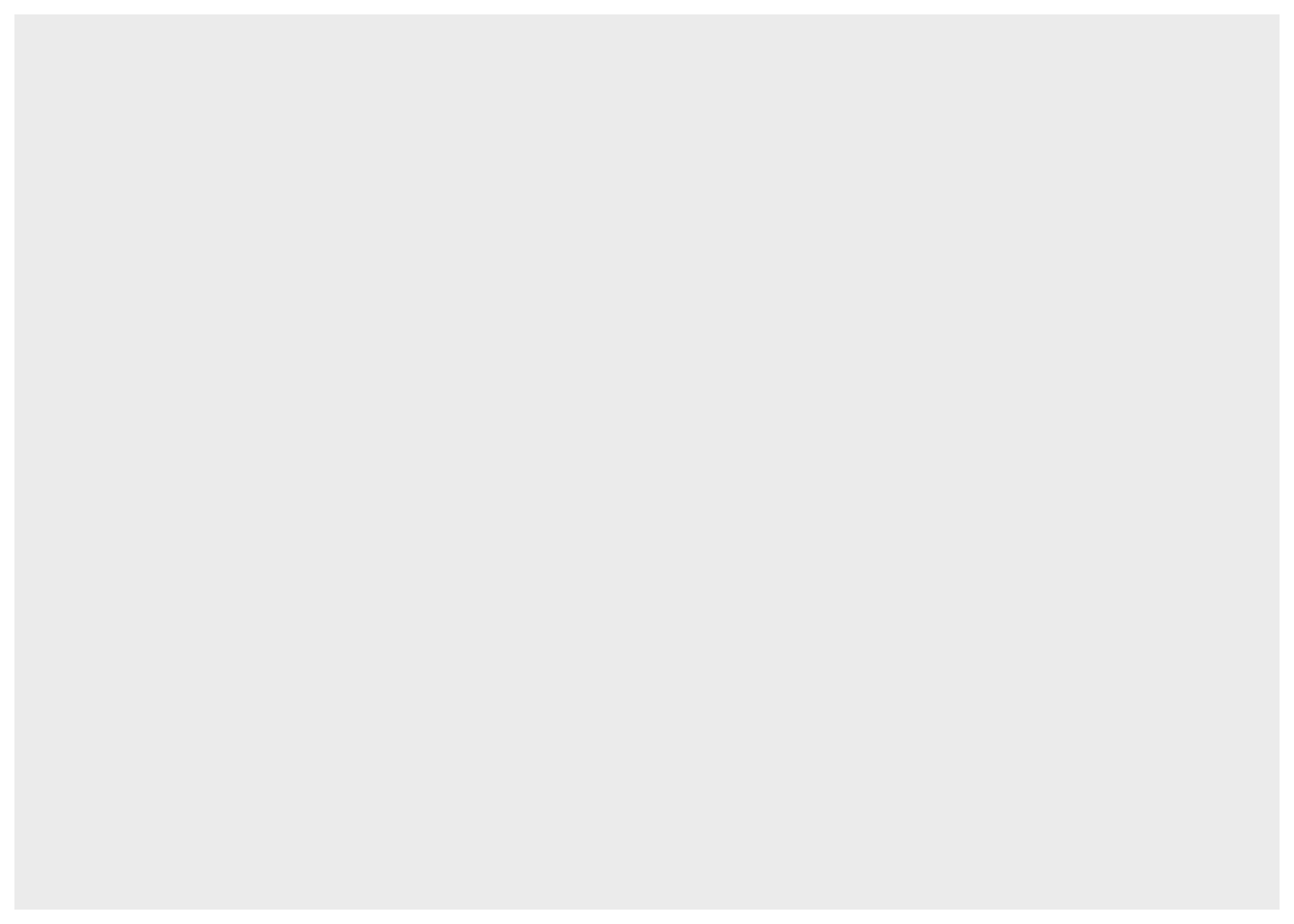1. Then we add the variables to be represented with the `aes()` function:
```ggplot(dat) + # data
aes(x = displ, y = hwy) # variables```1. Finally, we indicate the type of plot:
```ggplot(dat) + # data
aes(x = displ, y = hwy) + # variables
geom_point() # type of plot```You will also sometimes see the aesthetic elements (`aes()` with the variables) inside the `ggplot()` function in addition to the dataset:

```ggplot(mpg, aes(x = displ, y = hwy)) +
geom_point()```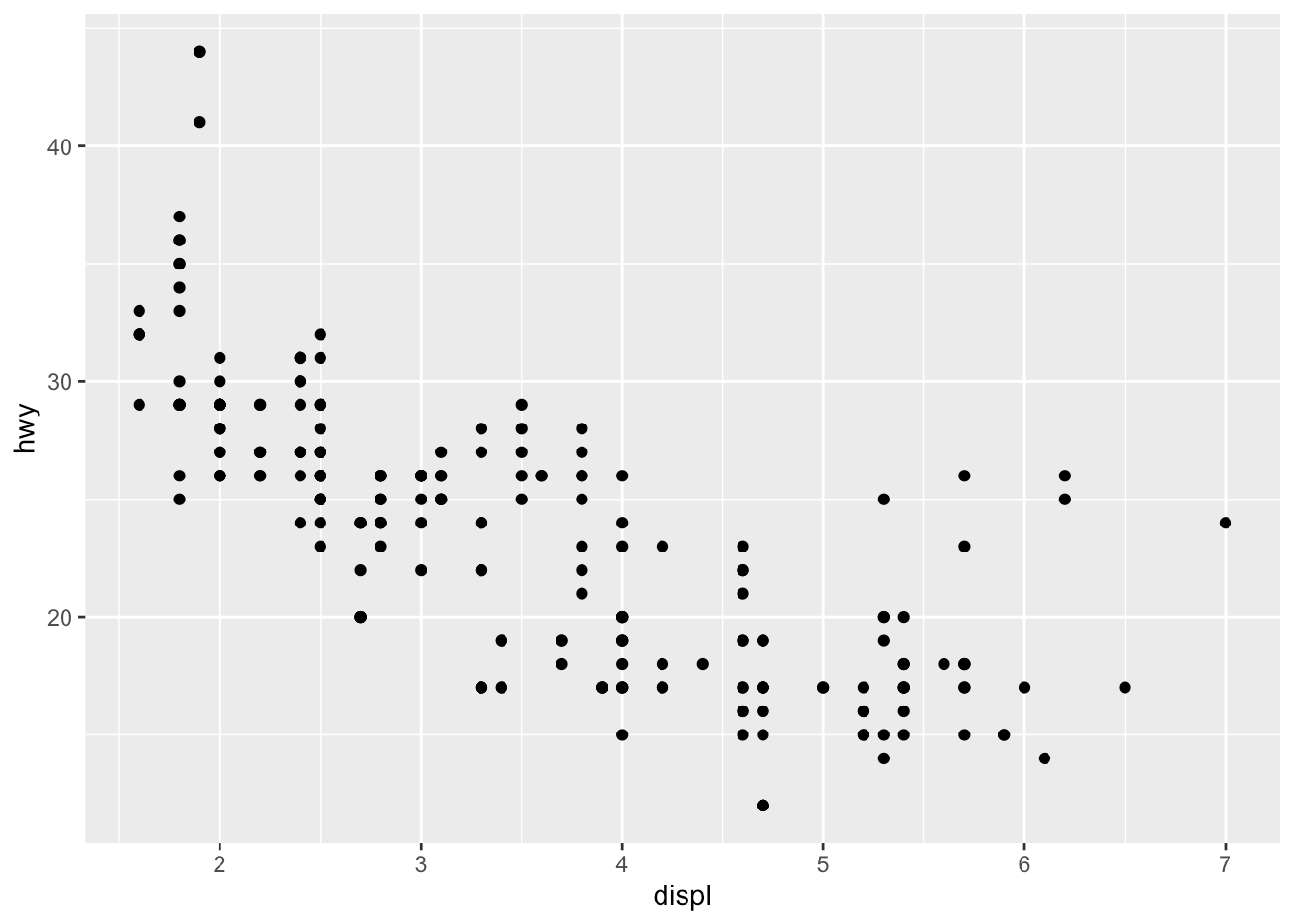This second method gives the exact same plot than the first method. I tend to prefer the first method over the second for better readability, but this is more a matter of taste so the choice is up to you.

## Line plot

Line plots, particularly useful in time series or finance, can be created similarly but by using `geom_line()`:

```ggplot(dat) +
aes(x = displ, y = hwy) +
geom_line()```## Combination of line and points

An advantage of `{ggplot2}` is the ability to combine several types of plots and its flexibility in designing it. For instance, we can add a line to a scatter plot by simply adding a layer to the initial scatter plot:

```ggplot(dat) +
aes(x = displ, y = hwy) +
geom_point() +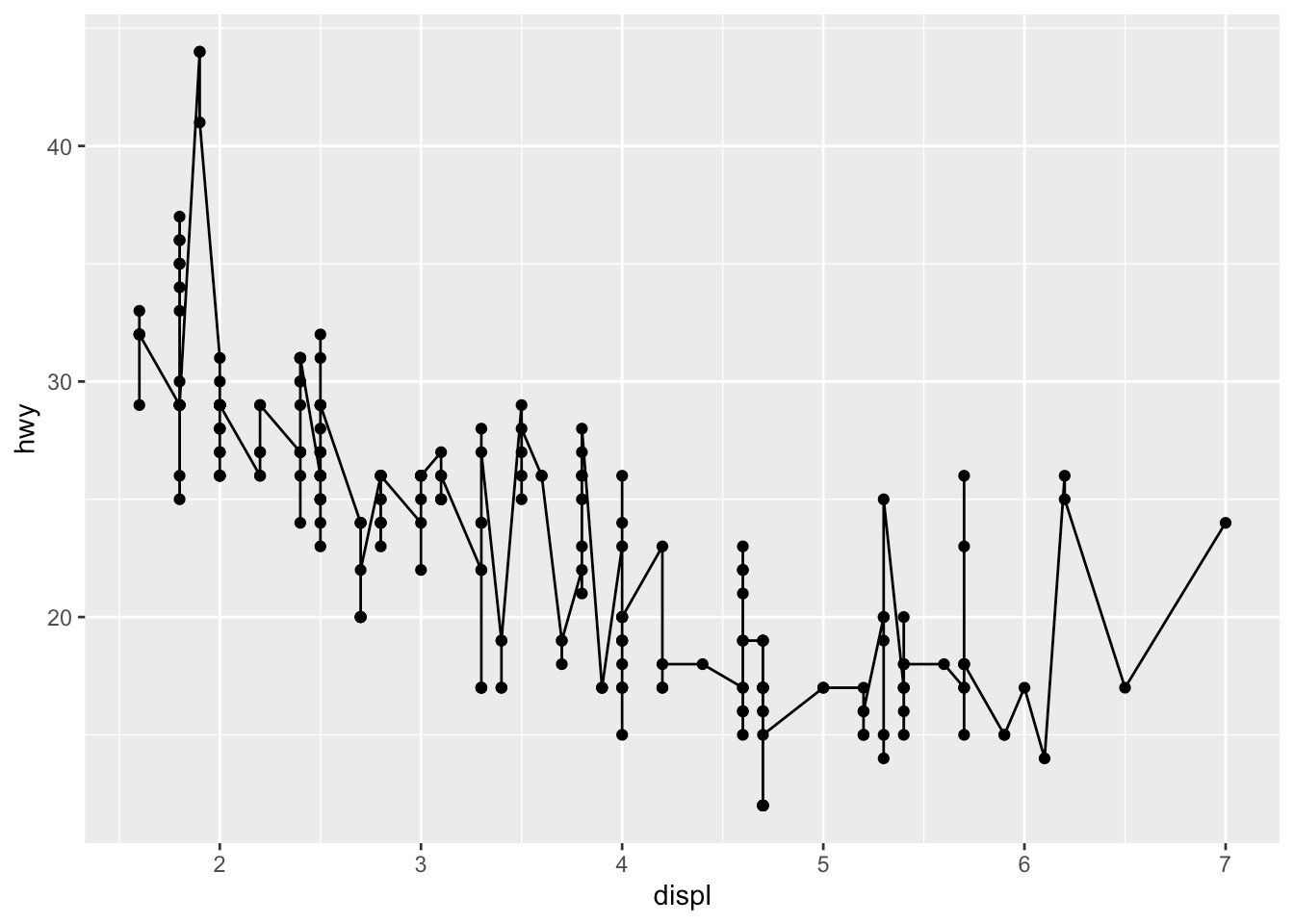## Histogram

A histogram (useful to visualize distributions) can be plotted using `geom_histogram()`:

```ggplot(dat) +
aes(x = hwy) +
geom_histogram()```By default, the number of bins is equal to 30. You can change this value using the `bins` argument inside the `geom_histogram()` function:

```ggplot(dat) +
aes(x = hwy) +
geom_histogram(bins = sqrt(nrow(dat)))```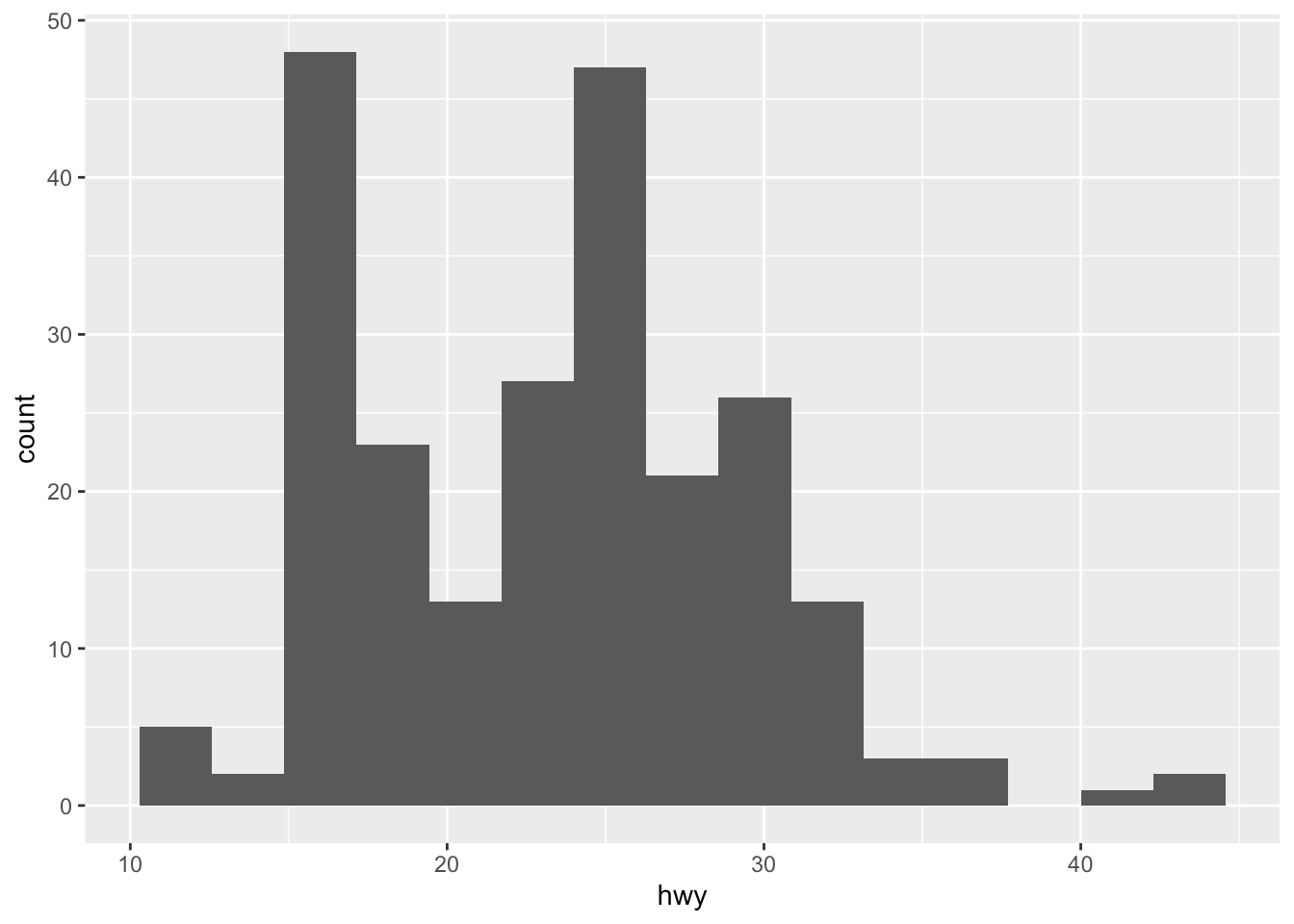Here I specify the number of bins to be equal to the square root of the number of observations (following Sturge’s rule) but you can specify any numeric value.

## Density plot

Density plots can be created using `geom_density()`:

```ggplot(dat) +
aes(x = hwy) +
geom_density()```## Combination of histogram and densities

We can also superimpose a histogram and a density curve on the same plot:

```ggplot(dat) +
aes(x = hwy, y = ..density..) +
geom_histogram() +
geom_density()```Or superimpose several densities:

```ggplot(dat) +
aes(x = hwy, color = drv, fill = drv) +
geom_density(alpha = 0.25) # add transparency```The argument `alpha = 0.25` has been added for some transparency. More information about this argument can be found in this section.

## Boxplot

A boxplot (also very useful to visualize distributions) can be plotted using `geom_boxplot()`:

```# Boxplot for one variable
ggplot(dat) +
aes(x = "", y = hwy) +
geom_boxplot()```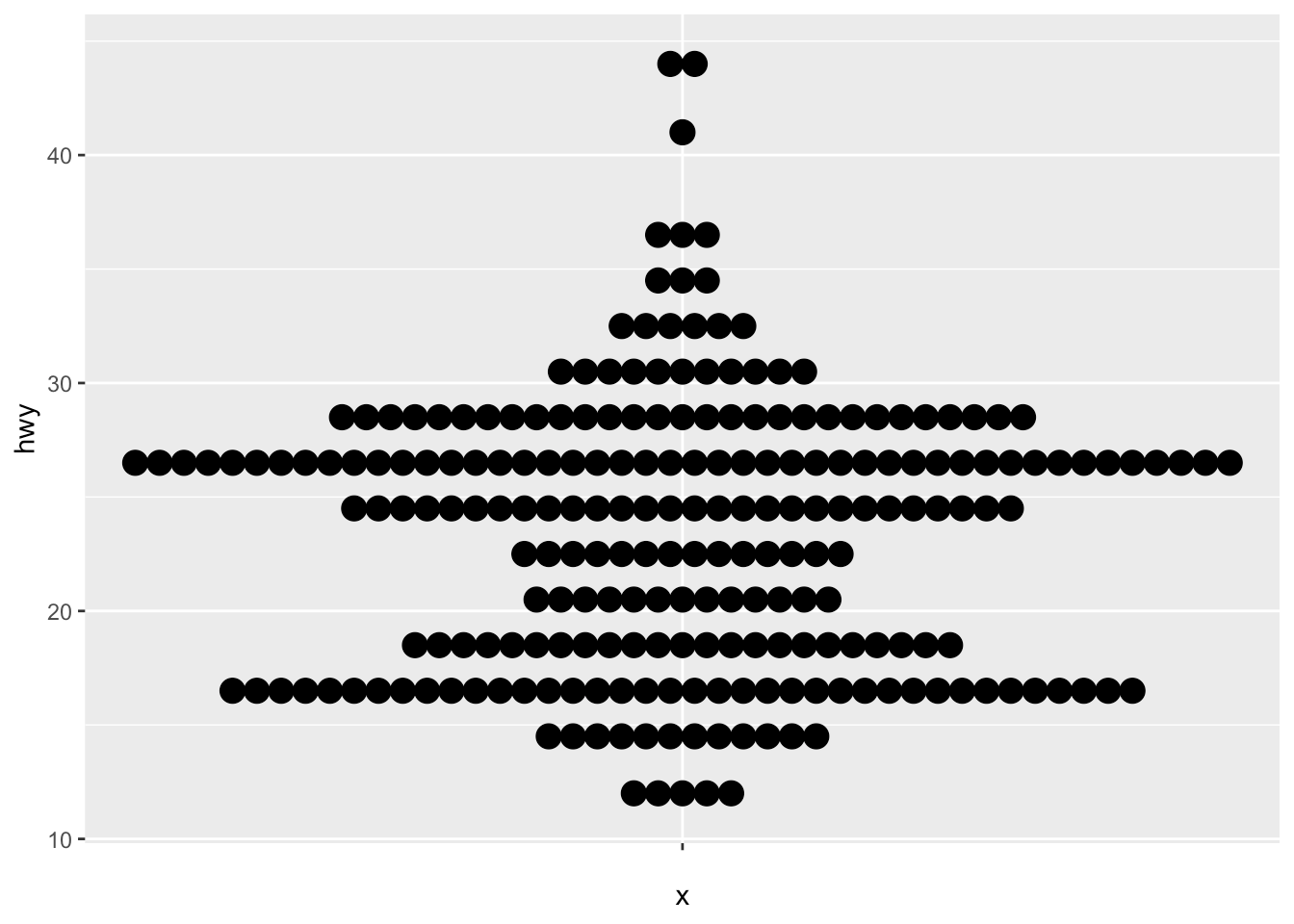```# Boxplot by factor
ggplot(dat) +
aes(x = drv, y = hwy) +
geom_boxplot()```It is also possible to plot the points on the boxplot with `geom_jitter()`, and to vary the width of the boxes according to the size (i.e., the number of observations) of each level with `varwidth = TRUE`:

```ggplot(dat) +
aes(x = drv, y = hwy) +
geom_boxplot(varwidth = TRUE) + # vary boxes width according to n obs.
geom_jitter(alpha = 0.25, width = 0.2) # adds random noise and limit its width```The `geom_jitter()` layer adds some random variation to each point in order to prevent them from overlapping (an issue known as overplotting).1 Moreover, the `alpha` argument adds some transparency to the points (see more in this section) to keep the focus on the boxes and not on the points.

Finally, it is also possible to divide boxplots into several panels according to the levels of a qualitative variable:

```ggplot(dat) +
aes(x = drv, y = hwy) +
geom_boxplot(varwidth = TRUE) + # vary boxes width according to n obs.
geom_jitter(alpha = 0.25, width = 0.2) + # adds random noise and limit its width
facet_wrap(~year) # divide into 2 panels```For a visually more appealing plot, it is also possible to use some colors for the boxes depending on the x variable:

```ggplot(dat) +
aes(x = drv, y = hwy, fill = drv) + # add color to boxes with fill
geom_boxplot(varwidth = TRUE) + # vary boxes width according to n obs.
geom_jitter(alpha = 0.25, width = 0.2) + # adds random noise and limit its width
facet_wrap(~year) + # divide into 2 panels
theme(legend.position = "none") # remove legend```In that case, it best to remove the legend as it becomes redundant. See more information about the legend in this section.

## Barplot

A barplot (useful to visualize qualitative variables) can be plotted using `geom_bar()`:

```ggplot(dat) +
aes(x = drv) +
geom_bar()```By default, the heights of the bars correspond to the observed frequencies for each level of the variable of interest (`drv` in our case).

Again, for a more appealing plot, we can add some colors to the bars with the `fill` argument:

```ggplot(dat) +
aes(x = drv, fill = drv) + # add colors to bars
geom_bar() +
theme(legend.position = "none") # remove legend```We can also create a barplot with two qualitative variables:

```ggplot(dat) +
aes(x = drv, fill = year) + # fill by years
geom_bar()```In order to compare proportions across groups, it is best to make each bar the same height using `position = "fill"`:

```ggplot(dat) +
geom_bar(aes(x = drv, fill = year), position = "fill")```To draw the bars next to each other for each group, use `position = "dodge"`:

```ggplot(dat) +
geom_bar(aes(x = drv, fill = year), position = "dodge")```## Further personalization

### Labels

The first things to personalize in a plot is the labels to make the plot more informative to the audience. We can easily add a title, subtitle, caption and edit axis titles with the `labs()` function:

```p <- ggplot(dat) +
aes(x = displ, y = hwy) +
geom_point()

p + labs(
title = "Fuel efficiency for 38 popular models of car",
subtitle = "Period 1999-2008",
caption = "Data: ggplot2::mpg. See more at www.statsandr.com",
x = "Engine displacement (litres)",
y = "Highway miles per gallon (mpg)"
)```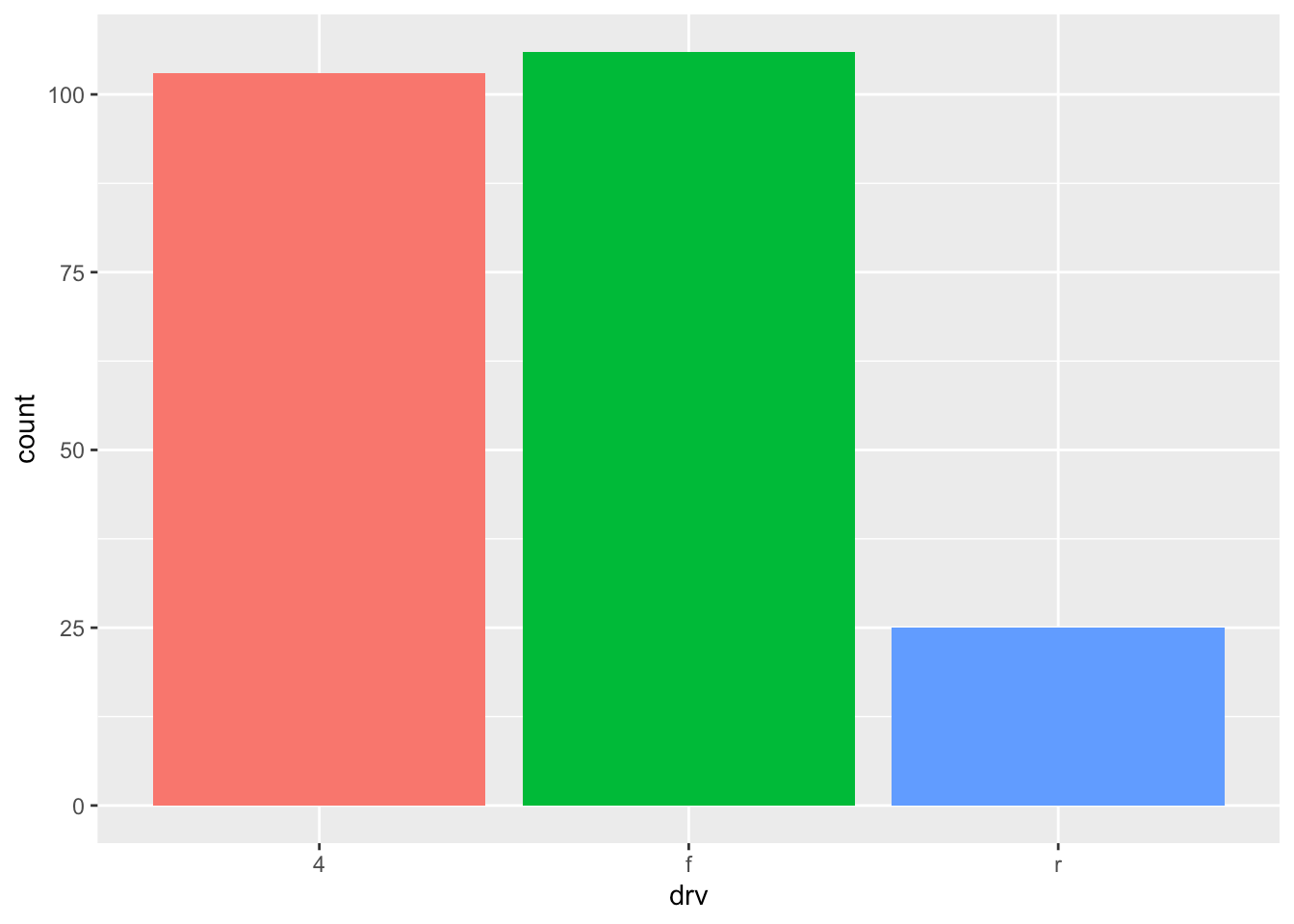As you can see in the above code, you can save one or more layers of the plot in an object for later use. This way, you can save your “main” plot, and add more layers of personalization until you get the desired output. Here we saved the main scatter plot in an object called `p` and we will refer to it for the subsequent personalizations.

### Axis ticks

Axis ticks can be adjusted using `scale_x_continuous()` and `scale_y_continuous()` for the x and y-axis, respectively:

```# Adjust ticks
p + scale_x_continuous(breaks = seq(from = 1, to = 7, by = 0.5)) + # x-axis
scale_y_continuous(breaks = seq(from = 10, to = 45, by = 5)) # y-axis```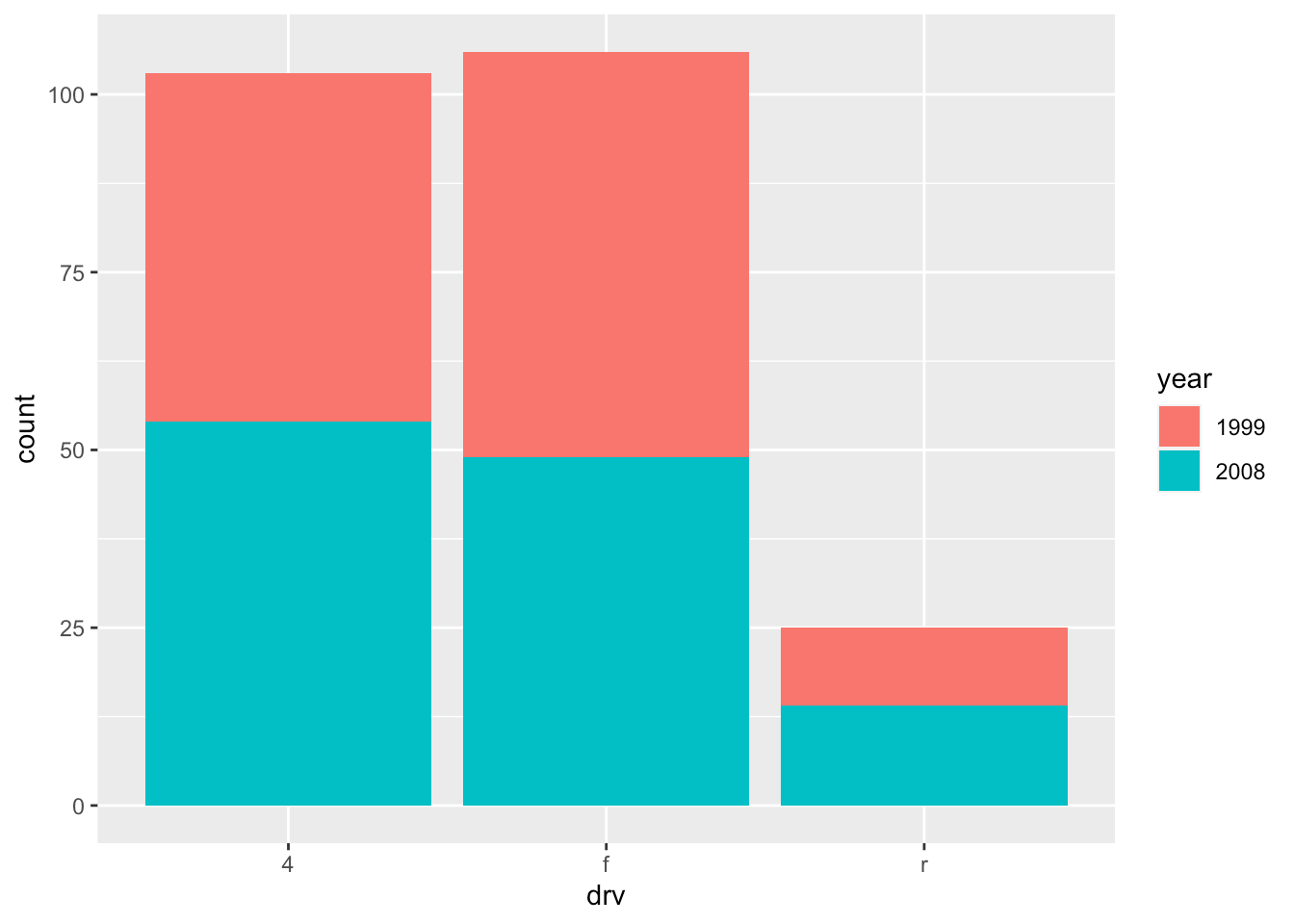### Log transformations

In some cases, it is useful to plot the log transformation of the variables. This can be done with the `scale_x_log10()` and `scale_y_log10()` functions:

```p + scale_x_log10() +
scale_y_log10()```### Limits

The most convenient way to control the limits of the plot is to use again the `scale_x_continuous()` and `scale_y_continuous()` functions in addition to the `limits` argument:

```p + scale_x_continuous(limits = c(3, 6)) +
scale_y_continuous(limits = c(20, 30))```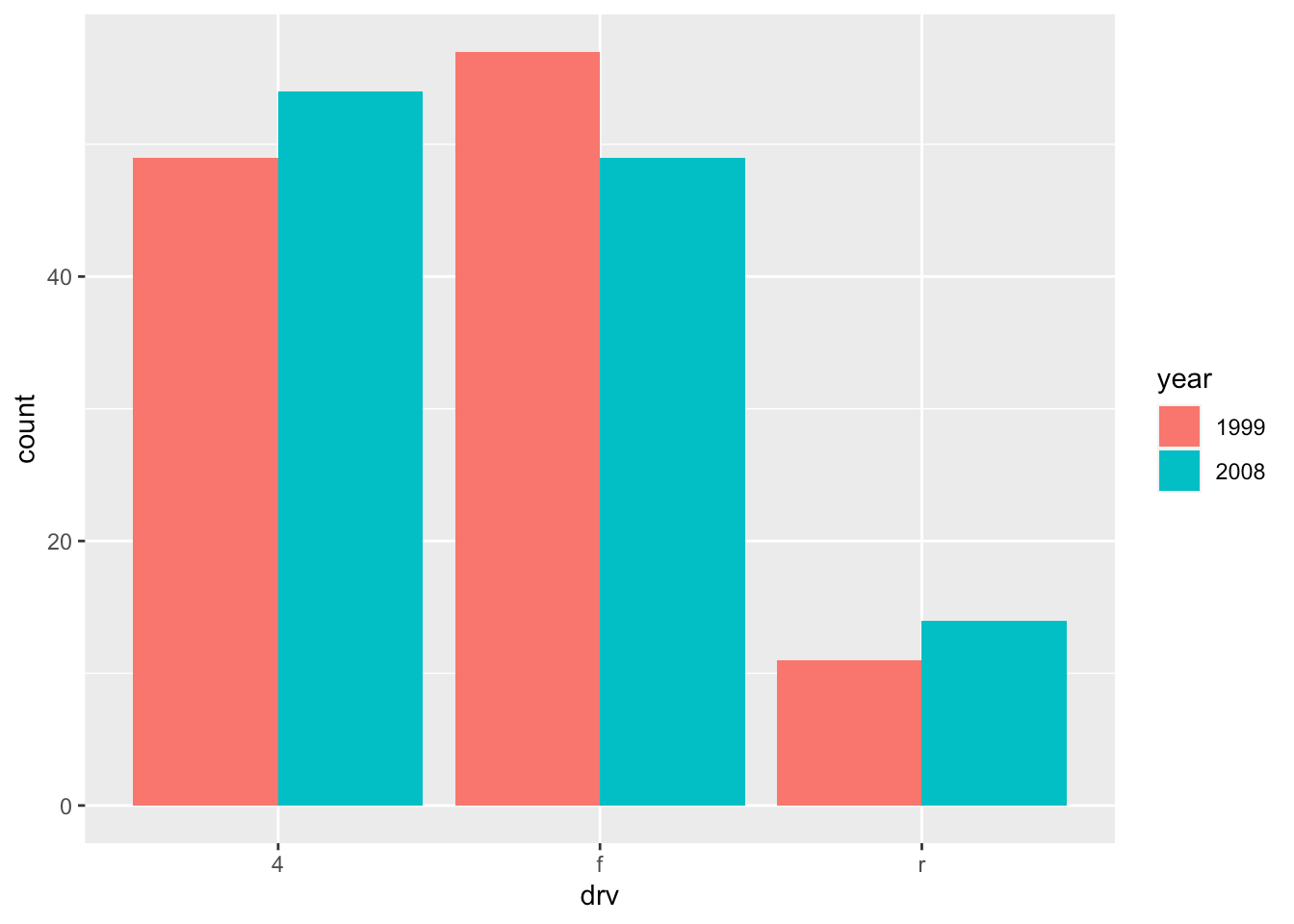It is also possible to simply take a subset of the dataset with the `subset()` or `filter()` function. See how to subset a dataset if you need a reminder.

### Legend

By default, the legend is located to the right side of the plot (when there is a legend to be displayed of course). To control the position of the legend, we need to use the `theme()` function in addition to the `legend.position` argument:

```p + aes(color = class) +
theme(legend.position = "top")```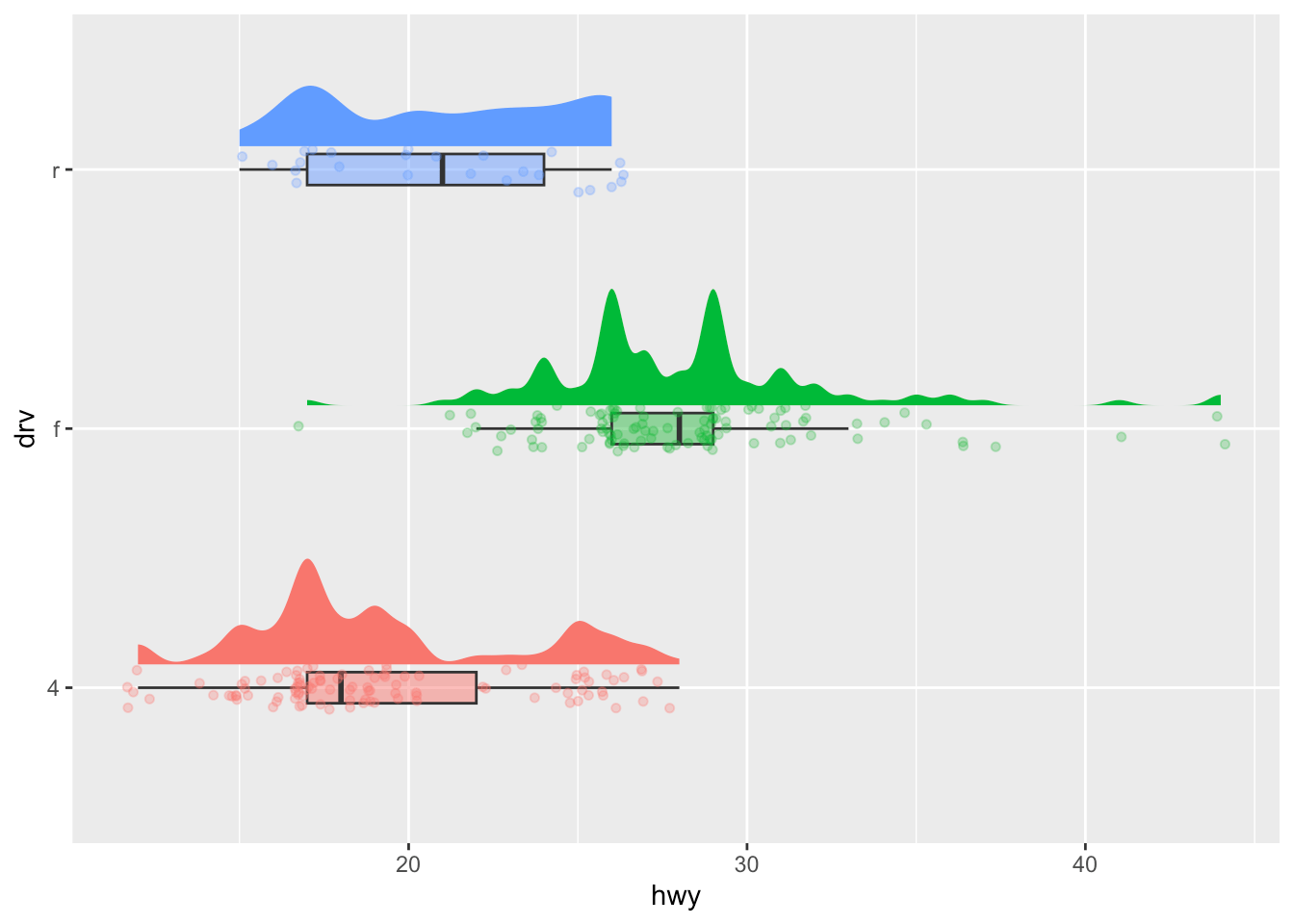Replace `"top"` by `"left"` or `"bottom"` to change its position and by `"none"` to remove it.

The title of the legend can be removed with `legend.title = element_blank()`:

```p + aes(color = class) +
theme(
legend.title = element_blank(),
legend.position = "bottom"
)```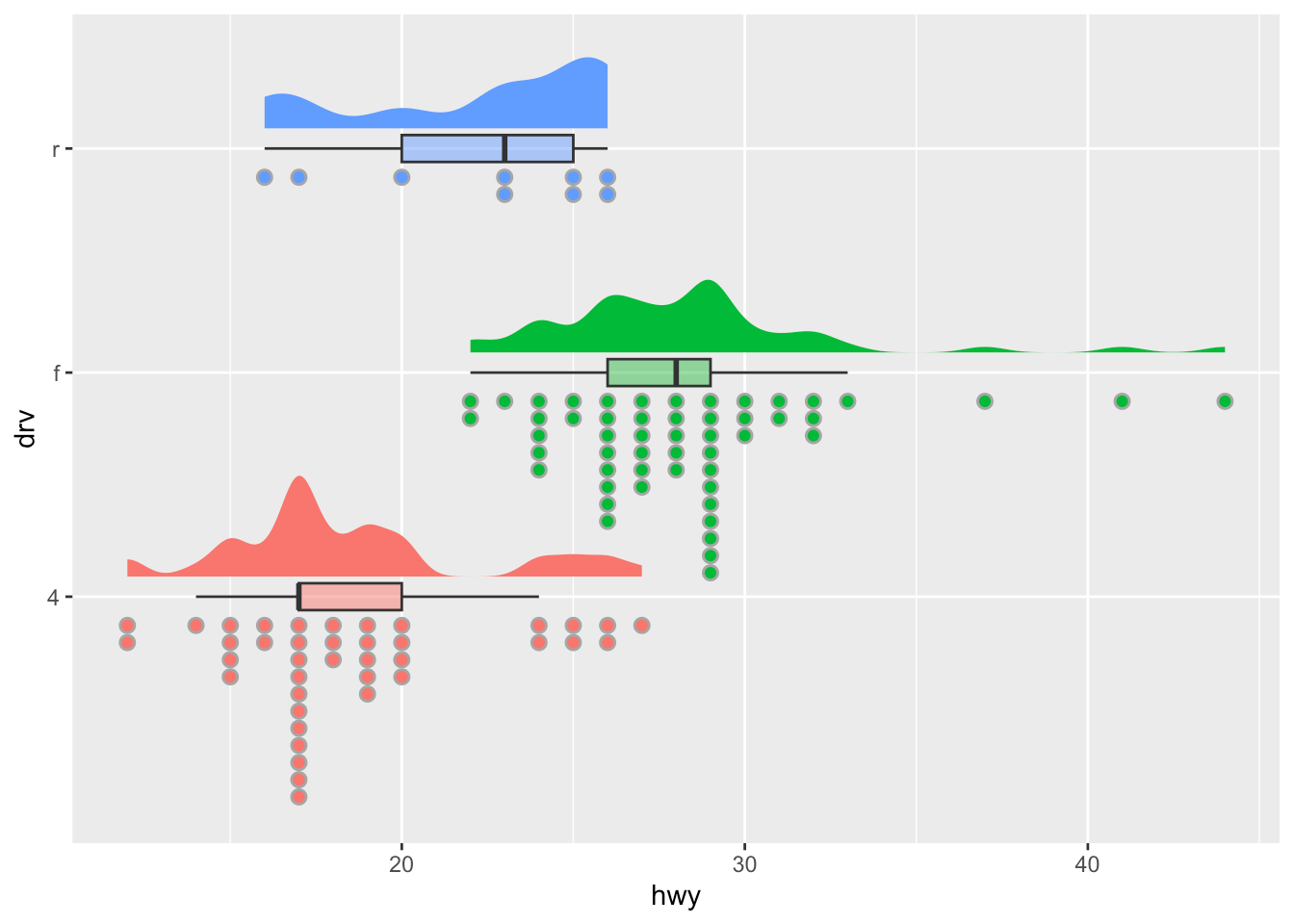The legend now appears at the bottom of the plot, without the legend title.

### Shape, color, size and transparency

There are a very large number of options to improve the quality of the plot or to add additional information. These include:

• shape,
• size,
• color, and
• alpha (transparency).

We can for instance change the shape of all points in a scatter plot by adding `shape` to `geom_point()`, or vary the shape according to the values taken by another variable (in that case, the `shape` argument must be inside `aes()`):2

```# Change shape of all points
ggplot(dat) +
aes(x = displ, y = hwy) +
geom_point(shape = 4)```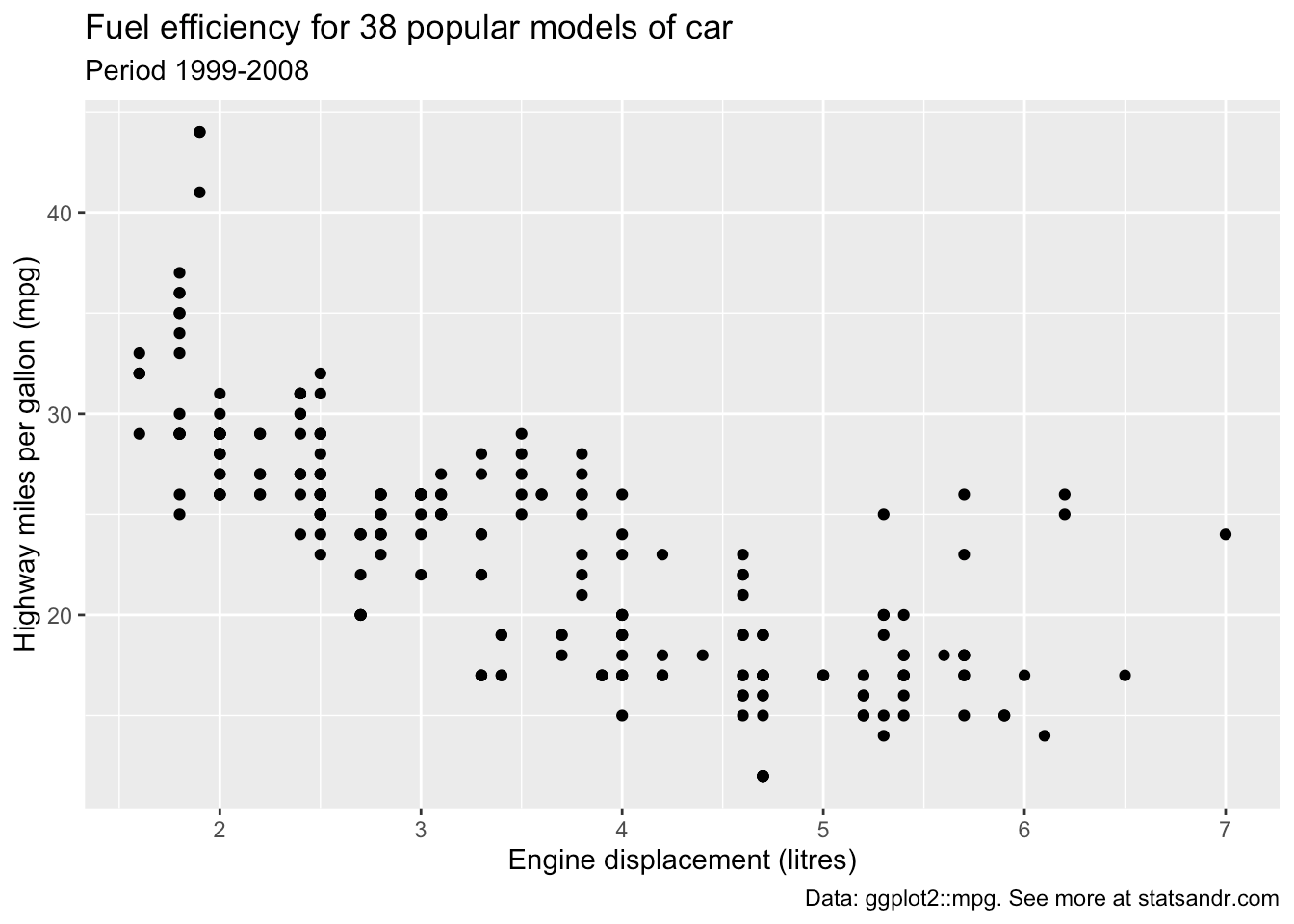```# Change shape of points based on a categorical variable
ggplot(dat) +
aes(x = displ, y = hwy, shape = drv) +
geom_point()```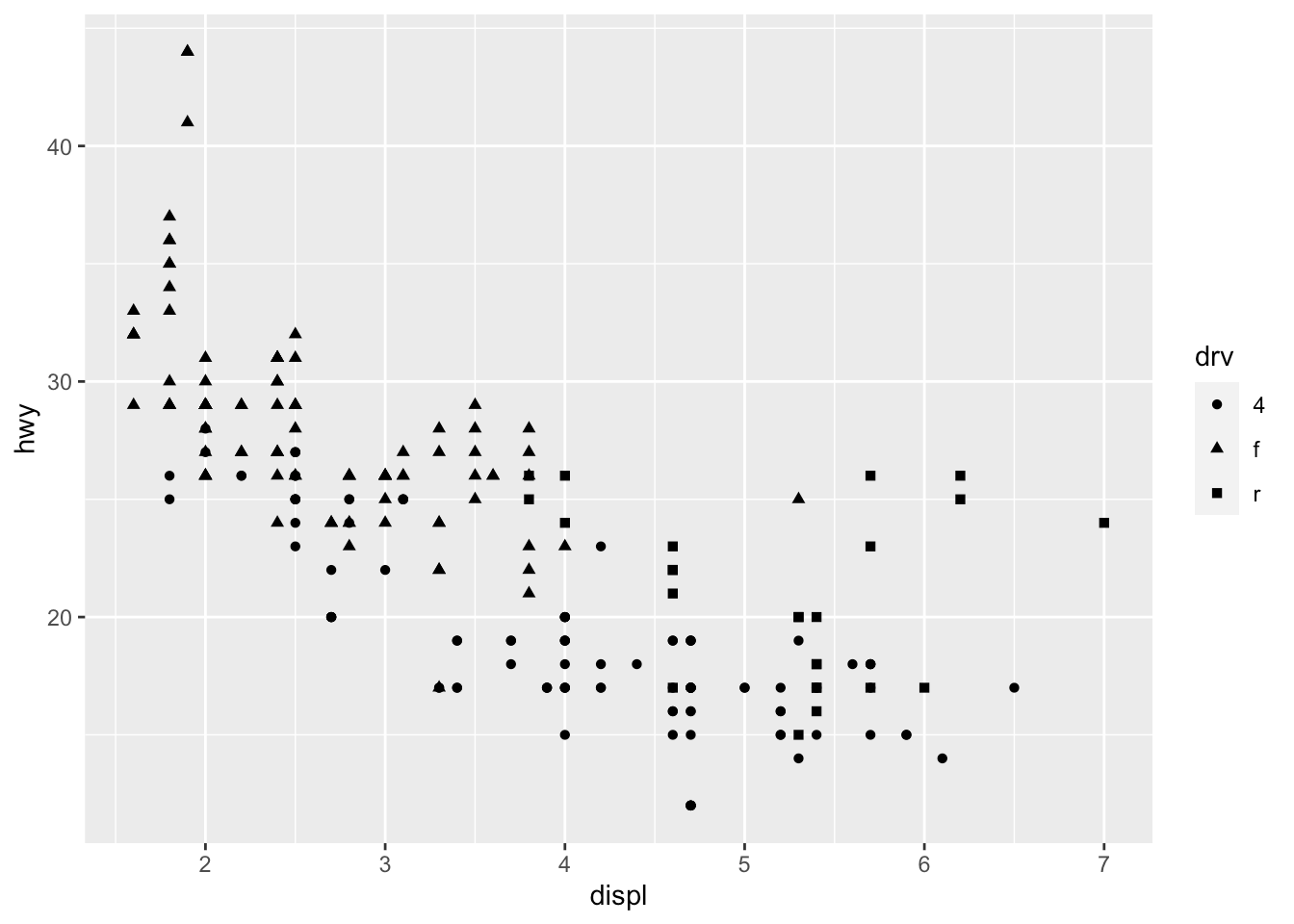Following the same principle, we can modify the color, size and transparency of the points based on a qualitative or quantitative variable. Here are some examples:

```p <- ggplot(dat) +
aes(x = displ, y = hwy) +
geom_point()

# Change color for all points
p + geom_point(color = "steelblue")```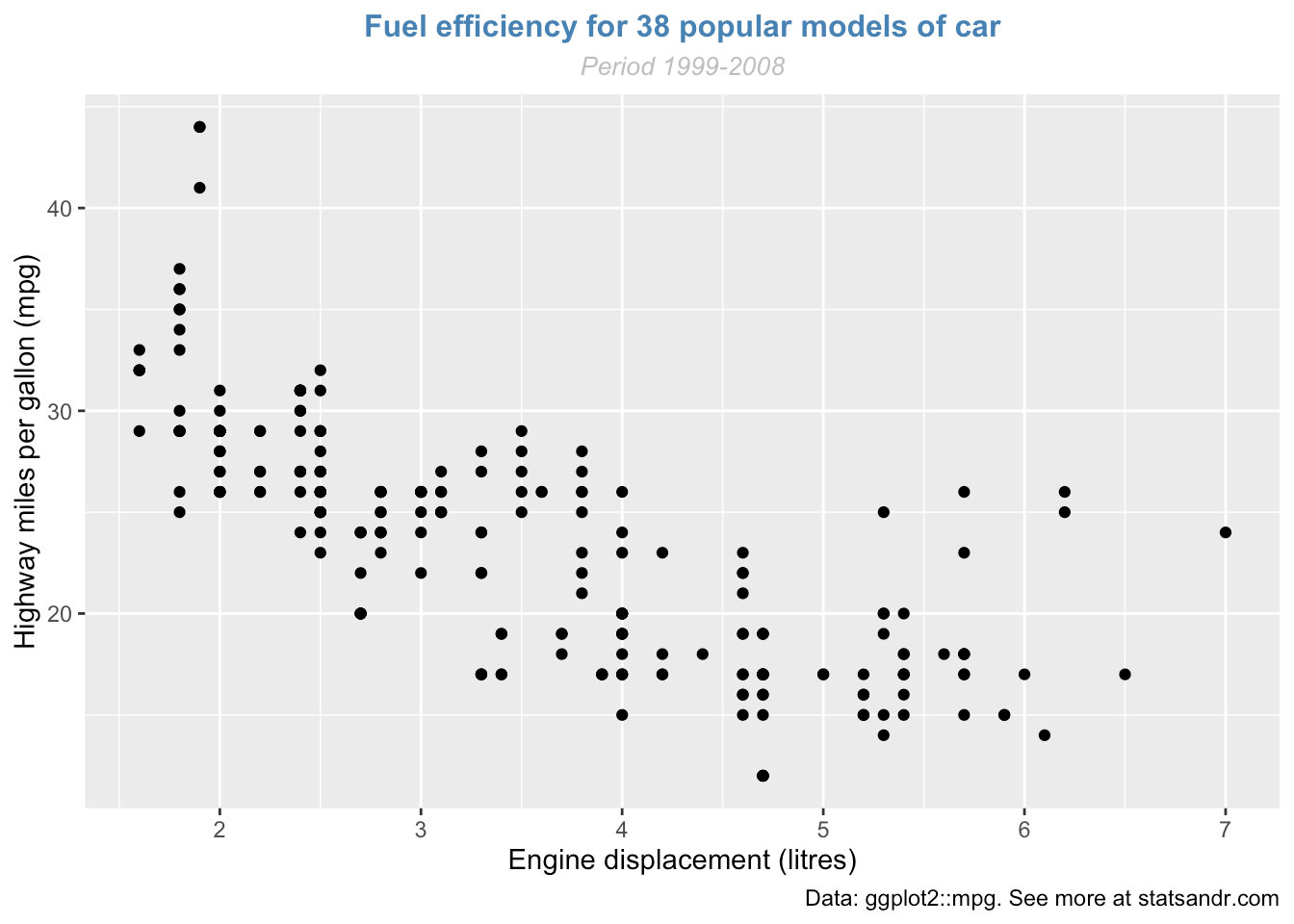```# Change color based on a qualitative variable
p + aes(color = drv)``````# Change color based on a quantitative variable
p + aes(color = cty)``````# Change color based on a criterion (median of cty variable)
p + aes(color = cty > median(cty))``````# Change size of all points
p + geom_point(size = 4)``````# Change size of points based on a quantitative variable
p + aes(size = cty)```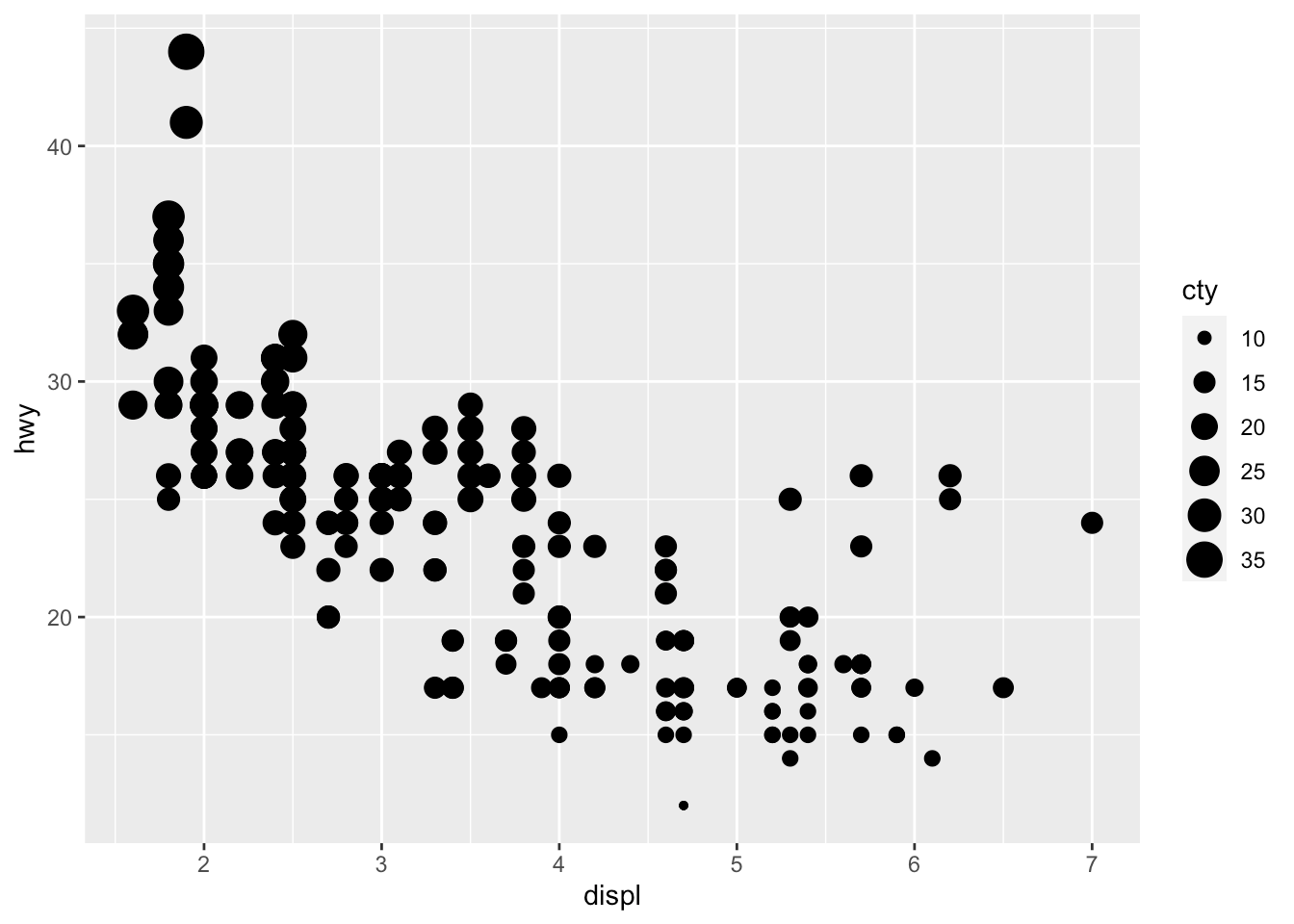```# Change transparency based on a quantitative variable
p + aes(alpha = cty)```We can of course mix several options (shape, color, size, alpha) to build more complex graphics:

```p + geom_point(size = 0.5) +
aes(color = drv, shape = year, alpha = cty)```### Smooth and regression lines

In a scatter plot, it is possible to add a smooth line fitted to the data:

`p + geom_smooth()`In the context of simple linear regression, it is often the case that the regression line is displayed on the plot. This can be done by adding `method = lm` (`lm` stands for linear model) in the `geom_smooth()` layer:

`p + geom_smooth(method = lm)`It is also possible to draw a regression line for each level of a categorical variable:

```p + aes(color = drv, shape = drv) +
geom_smooth(method = lm, se = FALSE)```The `se = FALSE` argument removes the confidence interval around the regression lines.

### Facets

`facet_grid` allows you to divide the same graphic into several panels according to the values of one or two qualitative variables:

```# According to one variable
p + facet_grid(. ~ drv)``````# According to 2 variables
p + facet_grid(drv ~ year)```It is then possible to add a regression line to each facet:

```p + facet_grid(. ~ drv) +
geom_smooth(method = lm)```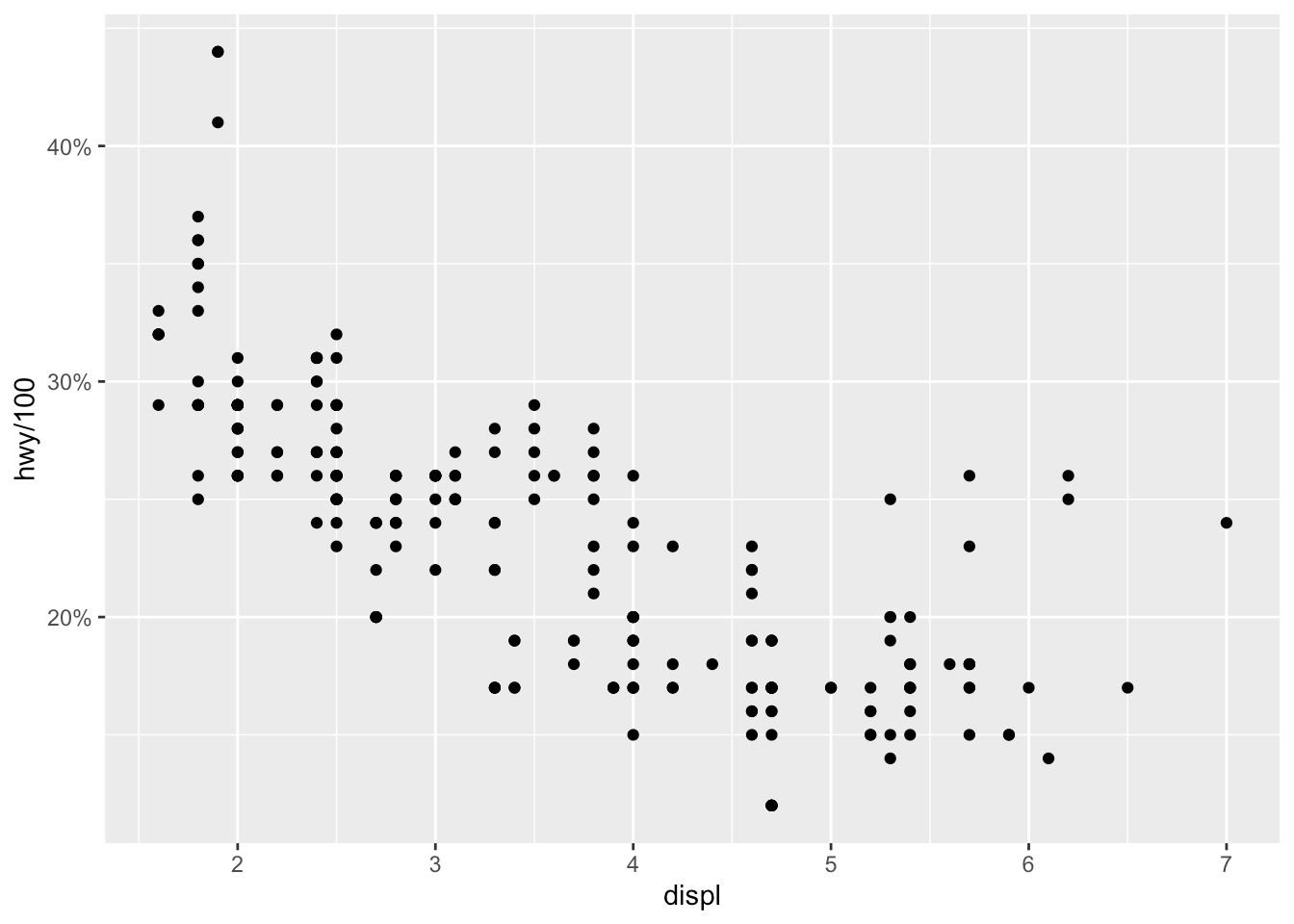`facet_wrap()` can also be used, as illustrated in this section.

### Themes

Several functions are available in the `{ggplot2}` package to change the theme of the plot. The most common themes after the default theme (i.e., `theme_gray()`) are the black and white (`theme_bw()`), minimal (`theme_minimal()`) and classic (`theme_classic()`) themes:

```# Black and white theme
p + theme_bw()```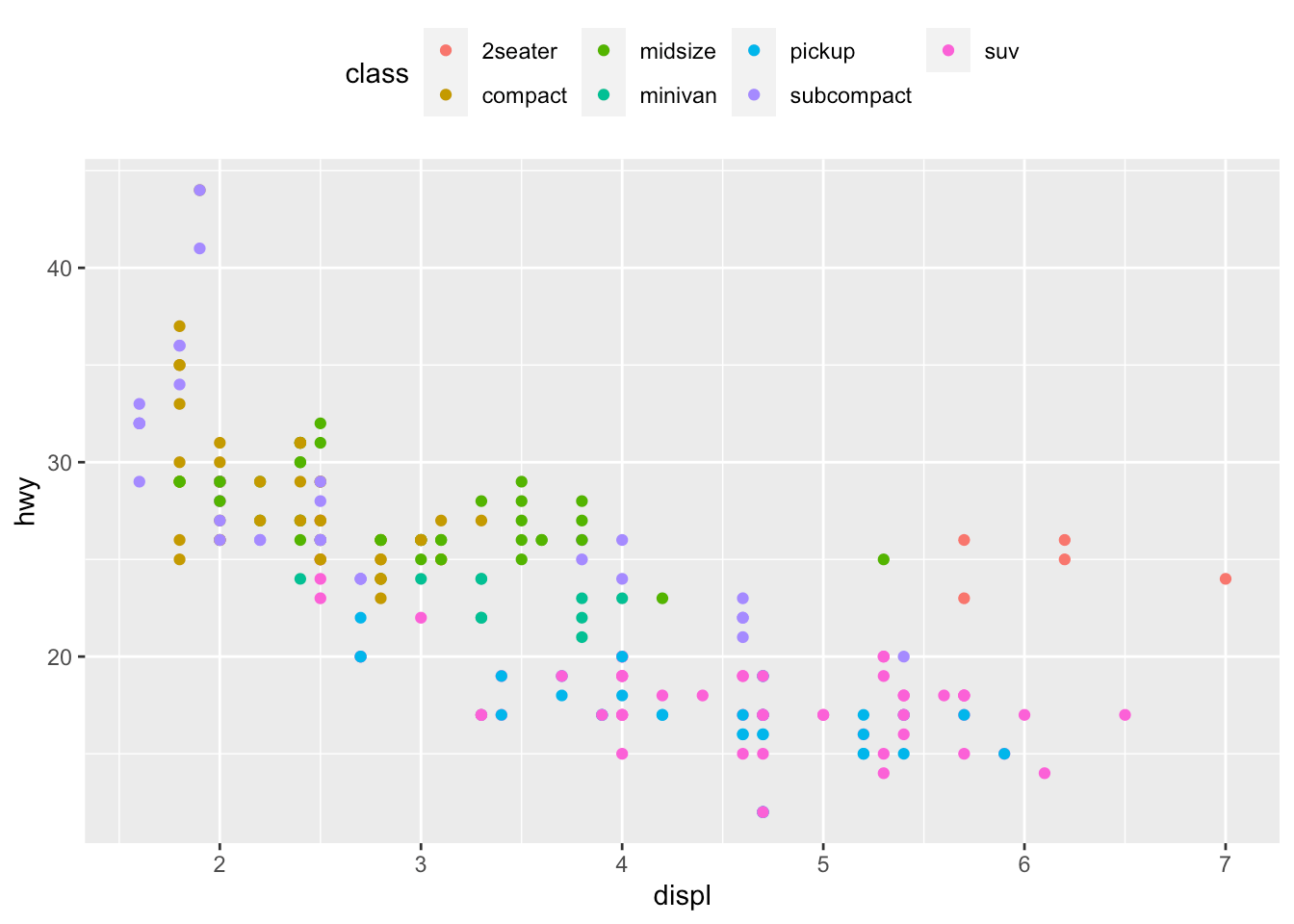```# Minimal theme
p + theme_minimal()``````# Classic theme
p + theme_classic()```I tend to use the minimal theme for most of my R Markdown reports as it brings out the patterns and points and not the layout of the plot, but again this is a matter of personal taste. See more themes at ggplot2.tidyverse.org/reference/ggtheme.html and in the `{ggthemes}` package.

In order to avoid having to change the theme for each plot you create, you can change the theme for the current R session using the `theme_set()` function as follows:

`theme_set(theme_minimal())`

### Interactive plot with `{plotly}`

You can easily make your plots created with `{ggplot2}` interactive with the `{plotly}` package:

```library(plotly)
ggplotly(p + aes(color = year))```

You can now hover over a point to display more information about that point. There is also the possibility to zoom in and out, to download the plot, to select some observations, etc. More information about `{plotly}` for R can be found here.

### Combine plots with `{patchwork}`

There are several ways to combine plots made in `{ggplot2}`. In my opinion, the most convenient way is with the `{patchwork}` package using symbols such as `+`, `/` and parentheses.

We first need to create some plots and save them:

```p_a <- ggplot(dat) +
aes(x = displ, y = hwy) +
geom_point()

p_b <- ggplot(dat) +
aes(x = hwy) +
geom_histogram()

p_c <- ggplot(dat) +
aes(x = drv, y = hwy) +
geom_boxplot()```

Now that we have 3 plots saved in our environment, we can combine them. To have plots next to each other simply use the `+` symbol:

```library(patchwork)
p_a + p_b + p_c```To display them above each other simply use the `/` symbol:

`p_a / p_b / p_c`And finally, to combine them above and next to each other, mix `+`, `/` and parentheses:

`p_a + p_b / p_c`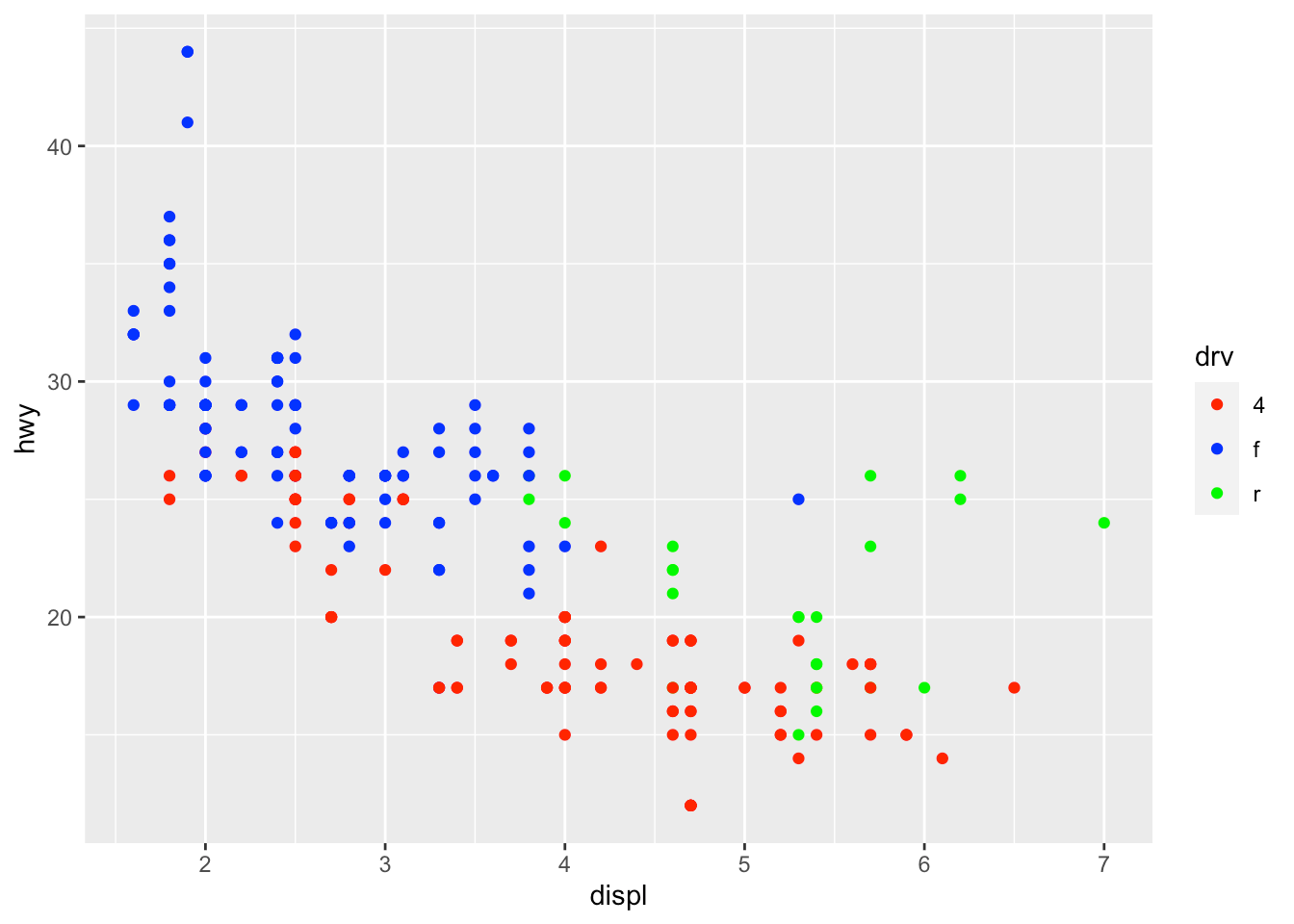`(p_a + p_b) / p_c`### Flip coordinates

Flipping coordinates of your plot is useful to create horizontal boxplots, or when labels of a variable are so long that they overlap each other on the x-axis. See with and without flipping coordinates below:

```# without flipping coordinates
p1 <- ggplot(dat) +
aes(x = class, y = hwy) +
geom_boxplot()

# with flipping coordinates
p2 <- ggplot(dat) +
aes(x = class, y = hwy) +
geom_boxplot() +
coord_flip()

library(patchwork)
p1 + p2 # left: without flipping, right: with flipping```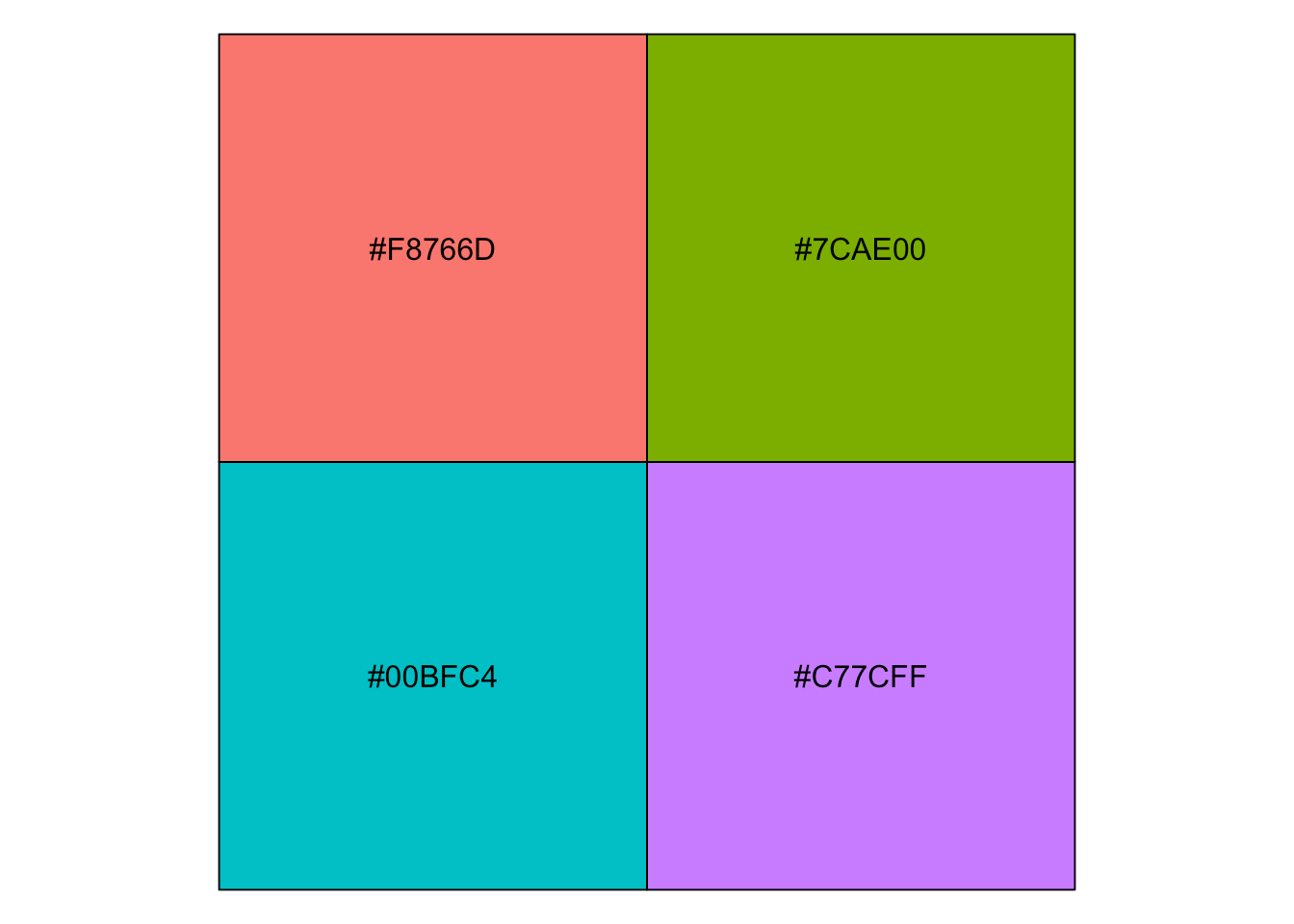This can be done with many types of plot, not only with boxplots. For instance, if a categorical variable has many levels or the labels are long, it is usually best to flip the coordinates for a better visual:

```ggplot(dat) +
aes(x = class) +
geom_bar() +
coord_flip()```### Save plot

The `ggsave()` function will save the most recent plot in your current working directory unless you specify a path to another folder:

```ggplot(dat) +
aes(x = displ, y = hwy) +
geom_point()

ggsave("plot1.pdf")```

# To go further

By now you have seen that `{ggplot2}` is a very powerful and complete package to create plots in R. This article illustrated only the tip of the iceberg, and you will find many tutorials on how to create more advanced plots and visualizations with `{ggplot2}` online. If you want to learn more than what is described in the present article, I highly recommend starting with:

Thanks for reading. I hope this article helped you to create your first plots with the `{ggplot2}` package. As a reminder, for simple graphs, it is sometimes easier to draw them via the {esquisse} addin. After some time, you will quickly learn how to create them by yourselves and in no time you will be able to build complex and sophisticated data visualizations.

As always, if you have a question or a suggestion related to the topic covered in this article, please add it as a comment so other readers can benefit from the discussion.

1. Use the `geom_jitter()` layer with caution because, although it makes a plot more revealing at large scales, it also makes it slightly less accurate at small scales since some randomness is added to the points.↩︎

2. There are (at the time of writing) 26 shapes accepted in the `shape` argument. See this documentation for all available shapes.↩︎